Share Results of Econometric Modeler App Session

This example shows how to share the results of an Econometric Modeler app session by:

• Exporting time series and model variables to the MATLAB® Workspace

• Generating MATLAB plain text and live functions to use outside the app

• Generating a report of your activities on time series and estimated models

During the session, the example transforms and plots data, runs statistical tests, and estimates a multiplicative seasonal ARIMA model. The data set Data_Airline.mat contains monthly counts of airline passengers.

Import Data into Econometric Modeler

At the command line, load the Data_Airline.mat data set.

At the command line, open the Econometric Modeler app.

econometricModeler

Alternatively, open the app from the apps gallery (see Econometric Modeler).

Import DataTable into the app:

1. On the Econometric Modeler tab, in the Import section, click.

2. In the Import Data dialog box, in the Import? column, select the check box for the DataTable variable.

3. Click .

The variable PSSG appears in the Time Series pane, its value appears in the Preview pane, and its time series plot appears in the Time Series Plot(PSSG) figure window.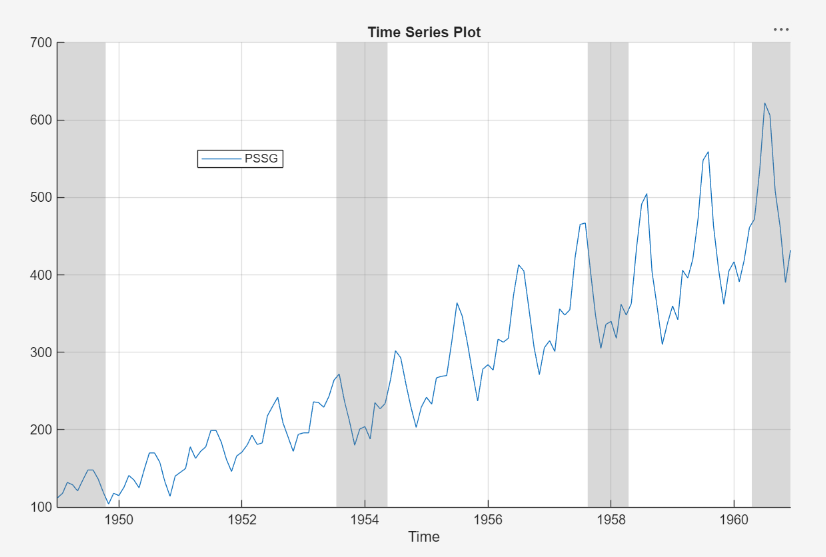The series exhibits a seasonal trend, serial correlation, and possible exponential growth. For an interactive analysis of serial correlation, see Detect Serial Correlation Using Econometric Modeler App.

Stabilize Series

Address the exponential trend by applying the log transform to PSSG.

1. In the Time Series pane, select PSSG.

2. On the Econometric Modeler tab, in the Transforms section, click .

The transformed variable PSSGLog appears in the Time Series pane, and its time series plot appears in the Time Series Plot(PSSGLog) figure window.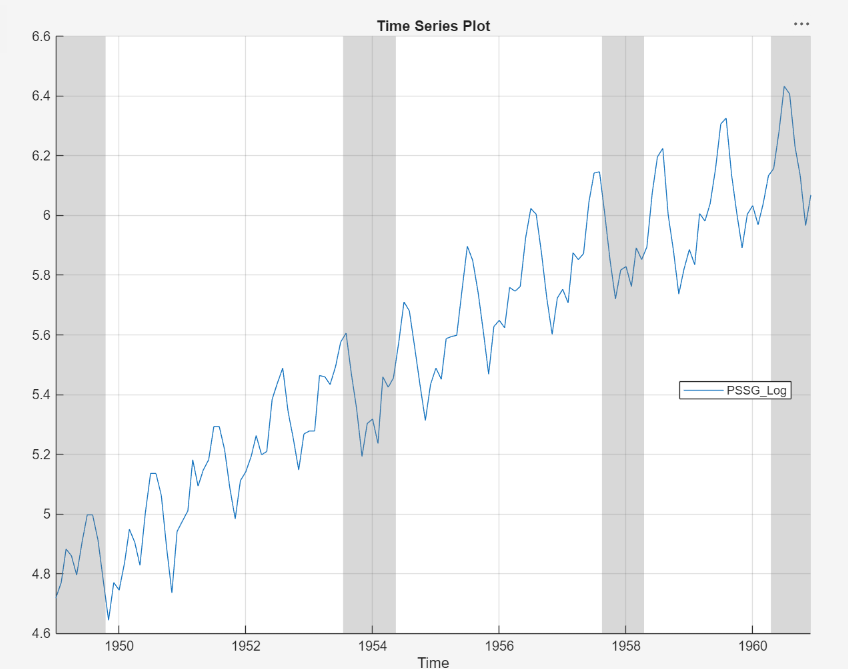The exponential growth appears to be removed from the series.

Address the seasonal trend by applying the 12th order seasonal difference. With PSSGLog selected in the Time Series pane, on the Econometric Modeler tab, in the Transforms section, set Seasonal to 12. Then, click .

The transformed variable PSSGLogSeasonalDiff appears in the Time Series pane, and its time series plot appears in the Time Series Plot(PSSGLogSeasonalDiff) figure window.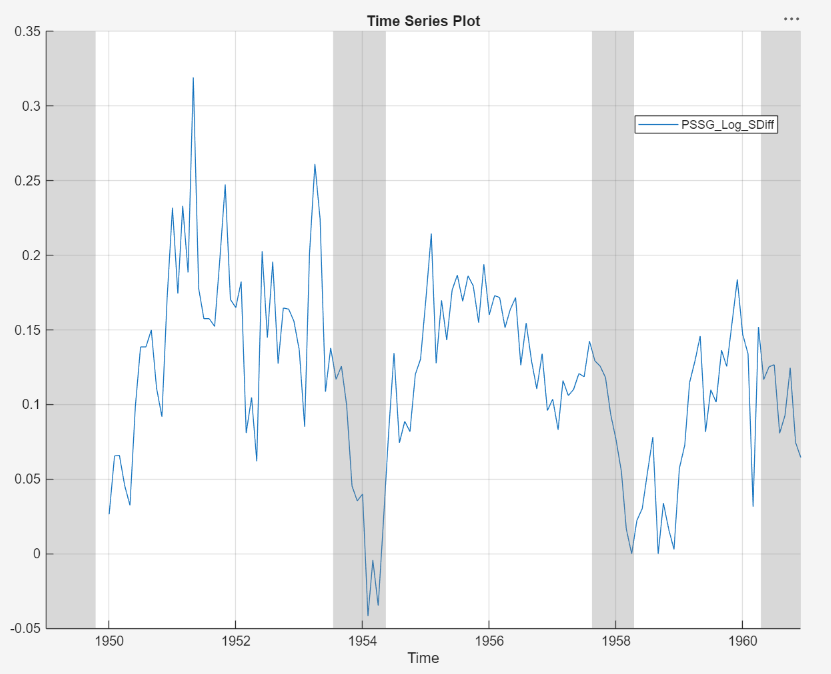The transformed series appears to have a unit root.

Test the null hypothesis that PSSGLogSeasonalDiff has a unit root by using the Augmented Dickey-Fuller test. Specify that the alternative is an AR(0) model, then test again specifying an AR(1) model. Adjust the significance level to 0.025 to maintain a total significance level of 0.05.

1. With PSSGLogSeasonalDiff selected in the Time Series pane, on the Econometric Modeler tab, in the Tests section, click > Augmented Dickey-Fuller Test.

2. On the ADF tab, in the Parameters section, set Significance Level to 0.025.

3. In the Tests section, click .

4. In the Parameters section, set Number of Lags to 1.

5. In the Tests section, click .

The test results appear in the Results table of the ADF(PSSGLogSeasonalDiff) document.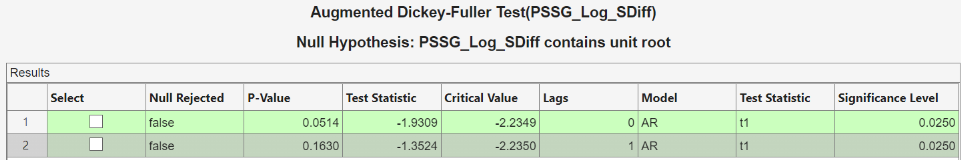Both tests fail to reject the null hypothesis that the series is a unit root process.

Address the unit root by applying the first difference to PSSGLogSeasonalDiff. With PSSGLogSeasonalDiff selected in the Time Series pane, click the Econometric Modeler tab. Then, in the Transforms section, click .

The transformed variable PSSGLogSeasonalDiffDiff appears in the Time Series pane, and its time series plot appears in the Time Series Plot(PSSGLogSeasonalDiffDiff) figure window.

In the Time Series pane, rename the PSSGLogSeasonalDiffDiff variable by clicking it twice to select its name and PSSGStable.

The app updates the names of all documents associated with the transformed series.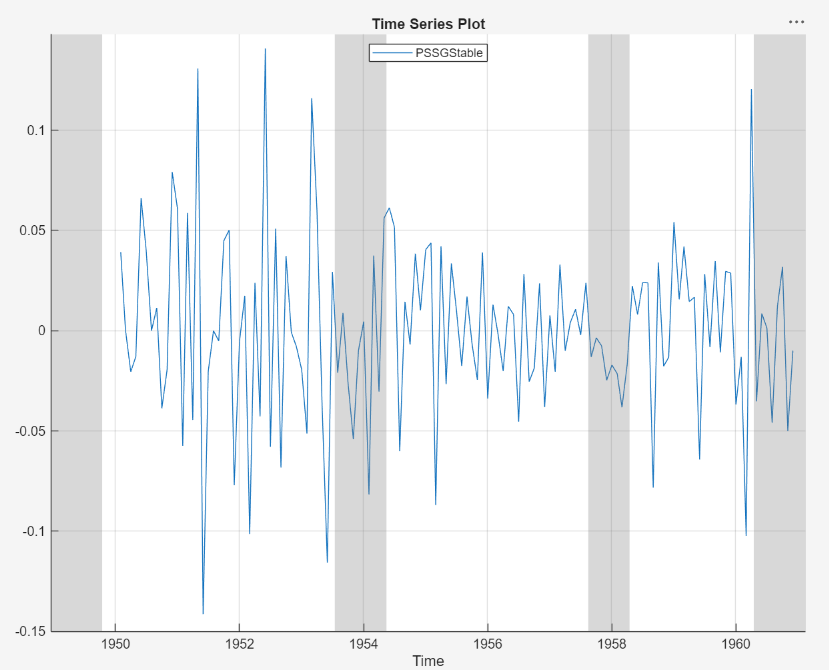Identify Model for Series

Determine the lag structure for a conditional mean model of the data by plotting the sample autocorrelation function (ACF) and partial autocorrelation function (PACF).

1. With PSSGStable selected in the Time Series pane, click the Plots tab, then click .

2. Show the first 50 lags of the ACF. On the ACF tab, set Number of Lags to 50.

3. Click the Plots tab, then click .

4. Show the first 50 lags of the PACF. On the PACF tab, set Number of Lags to 50.

5. Drag the ACF(PSSGStable) figure window above the PACF(PSSGStable) figure window.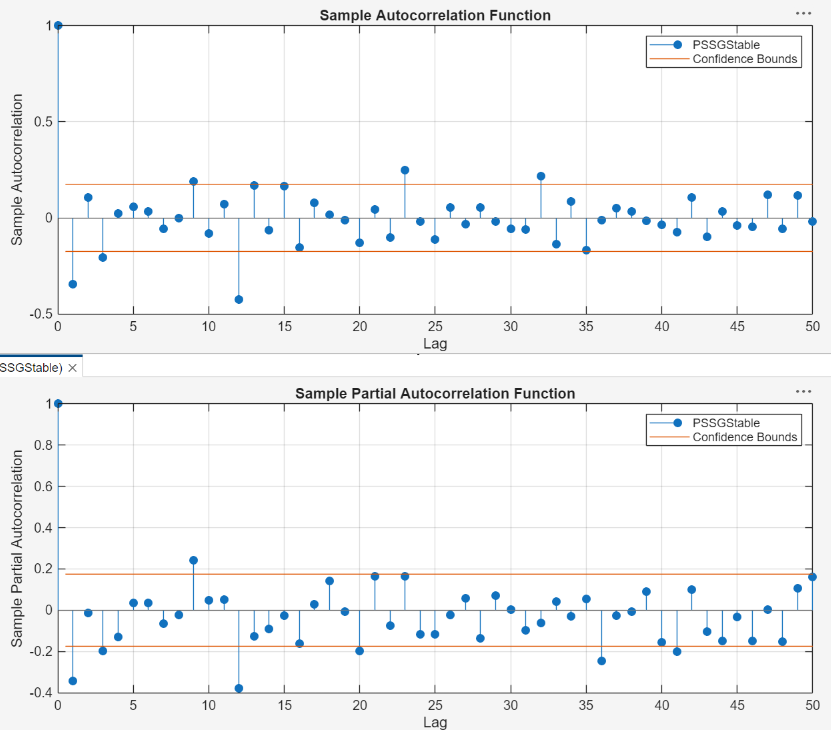According to , the autocorrelations in the ACF and PACF suggest that the following SARIMA(0,1,1)×(0,1,1)12 model is appropriate for PSSGLog.

$\left(1-L\right)\left(1-{L}^{12}\right){y}_{t}=\left(1+{\theta }_{1}L\right)\left(1+{\Theta }_{12}{L}^{12}\right){\epsilon }_{t}.$

Close all figure windows.

Specify and Estimate SARIMA Model

Specify the SARIMA(0,1,1)×(0,1,1)12 model.

1. In the Time Series pane, select the PSSGLog time series.

2. On the Econometric Modeler tab, in the Models section, click the arrow to display the models gallery.

3. In the models gallery, in the ARMA/ARIMA Models section, click .

4. In the SARIMA Model Parameters dialog box, on the Lag Order tab:

• Nonseasonal section

1. Set Degrees of Integration to 1.

2. Set Moving Average Order to 1.

3. Clear the Include Constant Term check box.

• Seasonal section

1. Set Period to 12 to indicate monthly data.

2. Set Moving Average Order to 1.

3. Select the Include Seasonal Difference check box.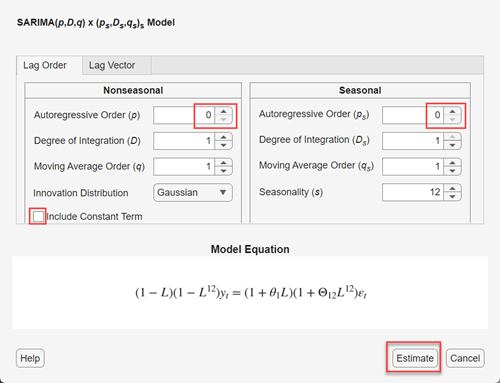5. Click .

The model variable SARIMA_PSSGLog appears in the Models pane, its value appears in the Preview pane, and its estimation summary appears in the Model Summary(SARIMA_PSSGLog) document.Export Variables to Workspace

Export PSSGLog, PSSGStable, and SARIMA_PSSGLog to the MATLAB Workspace.

1. On the Econometric Modeler tab, in the Export section, click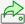.

2. In the Export Variables dialog box, select the Select check boxes for the PSSGLog and PSSGStable time series, and the SARIMA_PSSGLog model (if necessary). The app automatically selects the check boxes for all variables that are highlighted in the Time Series and Models panes.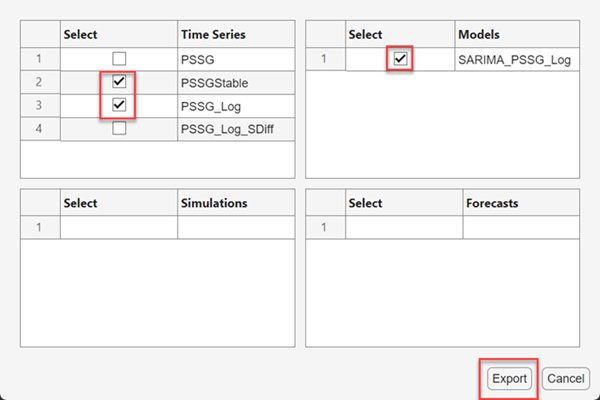3. Click .

At the command line, list all variables in the workspace.

whos
Name                  Size            Bytes  Class        Attributes

Data                144x1              1152  double
DataTable           144x1              3192  timetable
Description          22x54             2376  char
PSSGLog             144x1              1152  double
PSSGStable          144x1              1152  double
SARIMA_PSSGLog        1x1              7963  arima
dates               144x1              1152  double
series                1x1               162  cell

The contents of Data_Airline.mat, the numeric vectors PSSGLog and PSSGStable, and the estimated arima model object SARIMA_PSSGLog are variables in the workspace.

Forecast the next three years (36 months) of log airline passenger counts using SARIMA_PSSGLog. Specify the PSSGLog as presample data.

numObs = 36;
fPSSG = forecast(SARIMA_PSSGLog,numObs,'Y0',PSSGLog);

Plot the passenger counts and the forecasts.

fh = DataTable.Time(end) + calmonths(1:numObs);

figure;
plot(DataTable.Time,exp(PSSGLog));
hold on
plot(fh,exp(fPSSG));
legend('Airline Passenger Counts','Forecasted Counts',...
'Location','best')
title('Monthly Airline Passenger Counts, 1949-1963')
ylabel('Passenger counts')
hold off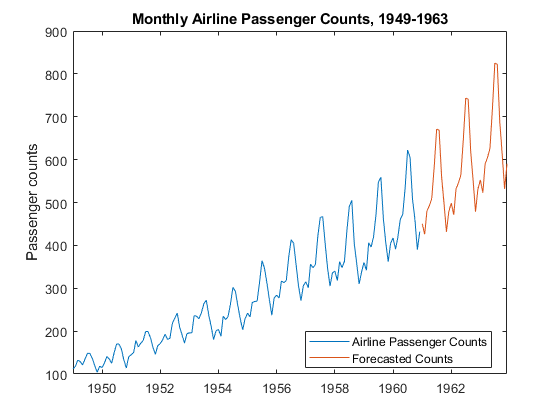Generate Plain Text Function from App Session

Generate a MATLAB function for use outside the app. The function returns the estimated model SARIMA_PSSGLog given DataTable.

1. In the Models pane of the app, select the SARIMA_PSSGLog model.

2. On the Econometric Modeler tab, in the Export section, click > Generate Function. The MATLAB Editor opens and contains a function named modelTimeSeries. The function accepts DataTable (the variable you imported in this session), transforms data, and returns the estimated SARIMA(0,1,1)×(0,1,1)12 model SARIMA_PSSGLog.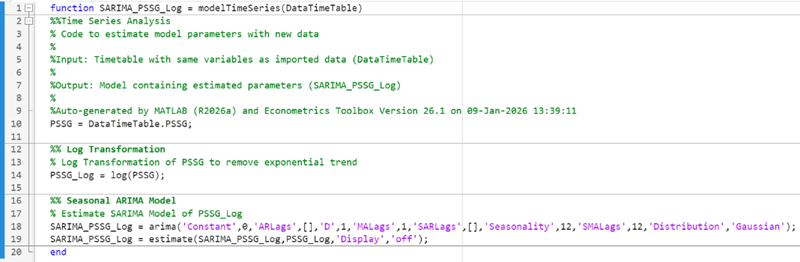3. On the Editor tab, click > Save.

4. Save the function to your current folder by clicking in the Select File for Save As dialog box.

At the command line, estimate the SARIMA(0,1,1)×(0,1,1)12 model by passing DataTable to modelTimeSeries.m. Name the model SARIMA_PSSGLog2. Compare the estimated model to SARIMA_PSSGLog.

SARIMA_PSSGLog2 = modelTimeSeries(DataTable);

summarize(SARIMA_PSSGLog)
summarize(SARIMA_PSSGLog2)
ARIMA(0,1,1) Model Seasonally Integrated with Seasonal MA(12) (Gaussian Distribution)

Effective Sample Size: 144
Number of Estimated Parameters: 3
LogLikelihood: 276.198
AIC: -546.397
BIC: -537.488

Value      StandardError    TStatistic      PValue
_________    _____________    __________    __________

Constant            0              0           NaN             NaN
MA{1}        -0.37716       0.066794       -5.6466      1.6364e-08
SMA{12}      -0.57238       0.085439       -6.6992      2.0952e-11
Variance    0.0012634     0.00012395        10.193      2.1406e-24

ARIMA(0,1,1) Model Seasonally Integrated with Seasonal MA(12) (Gaussian Distribution)

Effective Sample Size: 144
Number of Estimated Parameters: 3
LogLikelihood: 276.198
AIC: -546.397
BIC: -537.488

Value      StandardError    TStatistic      PValue
_________    _____________    __________    __________

Constant            0              0           NaN             NaN
MA{1}        -0.37716       0.066794       -5.6466      1.6364e-08
SMA{12}      -0.57238       0.085439       -6.6992      2.0952e-11
Variance    0.0012634     0.00012395        10.193      2.1406e-24

As expected, the models are identical.

Generate Live Function from App Session

Unlike a plain text function, a live function contains formatted text and equations that you can modify by using the Live Editor.

Generate a live function for use outside the app. The function returns the estimated model SARIMA_PSSGLog given DataTable.

1. In the Models pane of the app, select the SARIMA_PSSGLog model.

2. On the Econometric Modeler tab, in the Export section, click > Generate Live Function. The Live Editor opens and contains a function named modelTimeSeries. The function accepts DataTable (the variable you imported in this session), transforms data, and returns the estimated SARIMA(0,1,1)×(0,1,1)12 model SARIMA_PSSGLog.3. On the Live Editor tab, in the File section, click > Save.

4. Save the function to your current folder by clicking in the Select File for Save As dialog box.

At the command line, estimate the SARIMA(0,1,1)×(0,1,1)12 model by passing DataTable to modelTimeSeries.m. Name the model SARIMA_PSSGLog2. Compare the estimated model to SARIMA_PSSGLog.

SARIMA_PSSGLog2 = modelTimeSeries(DataTable);

summarize(SARIMA_PSSGLog)
summarize(SARIMA_PSSGLog2)
ARIMA(0,1,1) Model Seasonally Integrated with Seasonal MA(12) (Gaussian Distribution)

Effective Sample Size: 144
Number of Estimated Parameters: 3
LogLikelihood: 276.198
AIC: -546.397
BIC: -537.488

Value      StandardError    TStatistic      PValue
_________    _____________    __________    __________

Constant            0              0                        NaN
MA{1}        -0.37716       0.066794       -5.6466      1.6364e-08
SMA{12}      -0.57238       0.085439       -6.6992      2.0952e-11
Variance    0.0012634     0.00012395        10.193      2.1406e-24

ARIMA(0,1,1) Model Seasonally Integrated with Seasonal MA(12) (Gaussian Distribution)

Effective Sample Size: 144
Number of Estimated Parameters: 3
LogLikelihood: 276.198
AIC: -546.397
BIC: -537.488

Value      StandardError    TStatistic      PValue
_________    _____________    __________    __________

Constant            0              0           NaN             NaN
MA{1}        -0.37716       0.066794       -5.6466      1.6364e-08
SMA{12}      -0.57238       0.085439       -6.6992      2.0952e-11
Variance    0.0012634     0.00012395        10.193      2.1406e-24

As expected, the models are identical.

Generate Report

Generate a PDF report of all your actions on the PSSGLog and PSSGStable time series, and the SARIMA_PSSGLog model.

1. On the Econometric Modeler tab, in the Export section, click > Generate Report.

2. In the Select Variables for Report dialog box, select the Select check boxes for the PSSGLog and PSSGStable time series, and the SARIMA_PSSGLog model (if necessary). The app automatically selects the check boxes for all variables that are highlighted in the Time Series and Models panes.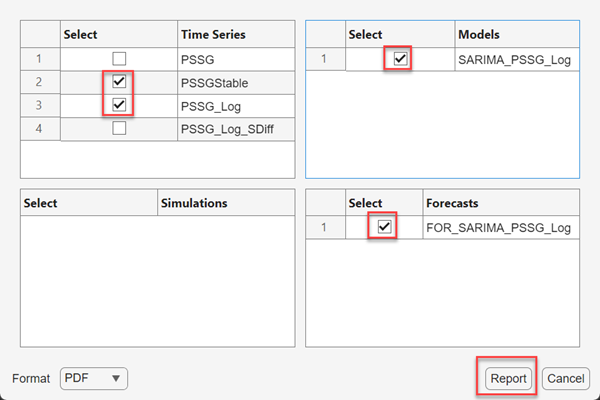3. Click .

4. In the Select File to Write dialog box, navigate to the C:\MyData folder.

5. In the File name box, type SARIMAReport.

6. Click .

The app publishes the code required to create PSSGLog, PSSGStable, and SARIMA_PSSGLog in the PDF C:\MyData\SARIMAReport.pdf. The report includes:

• Plots that include the selected time series

• Descriptions of transformations applied to the selected time series

• Results of statistical tests conducted on the selected time series

• Estimation summaries of the selected models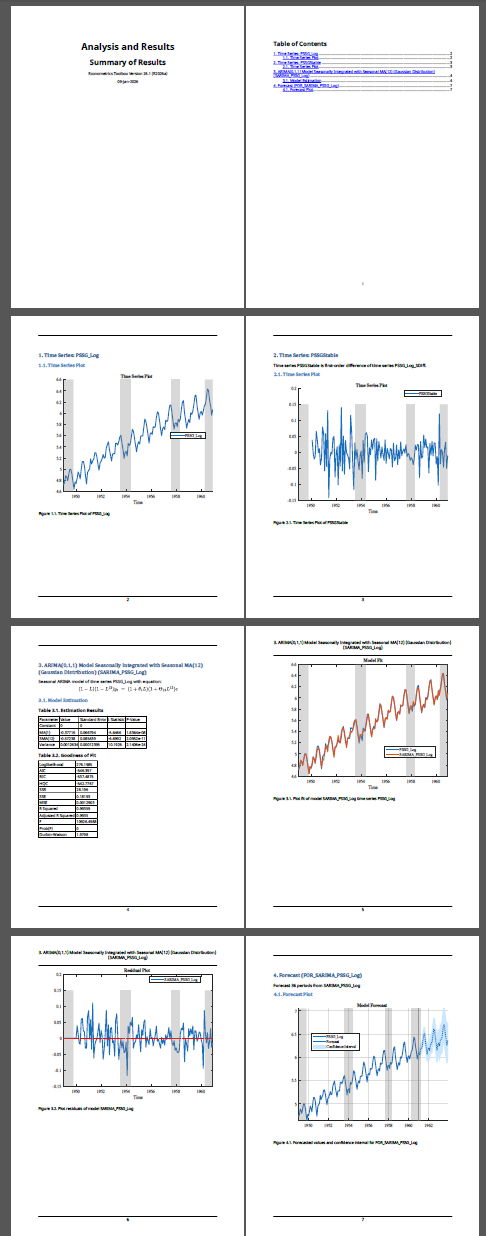Box, George E. P., Gwilym M. Jenkins, and Gregory C. Reinsel. Time Series Analysis: Forecasting and Control. 3rd ed. Englewood Cliffs, NJ: Prentice Hall, 1994.